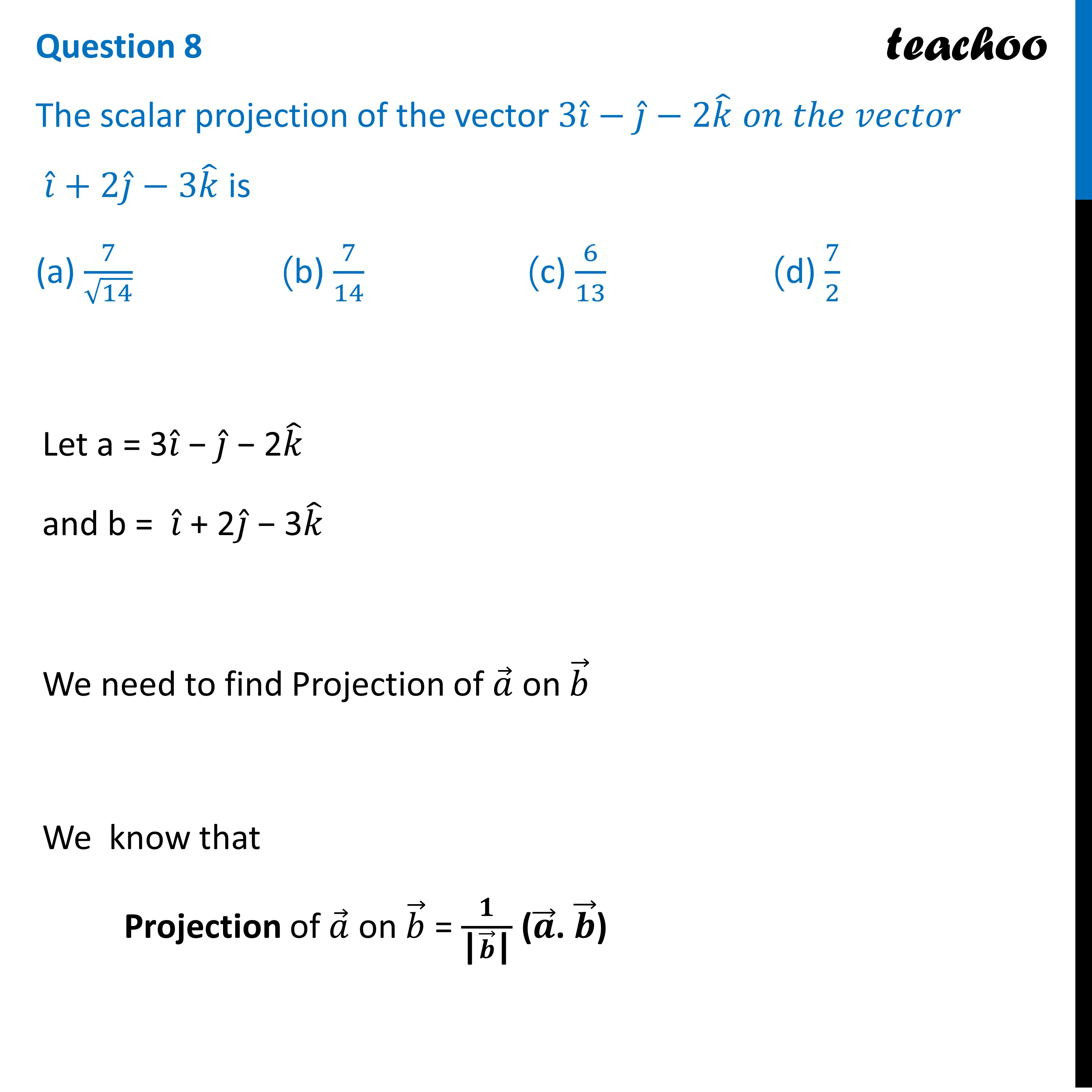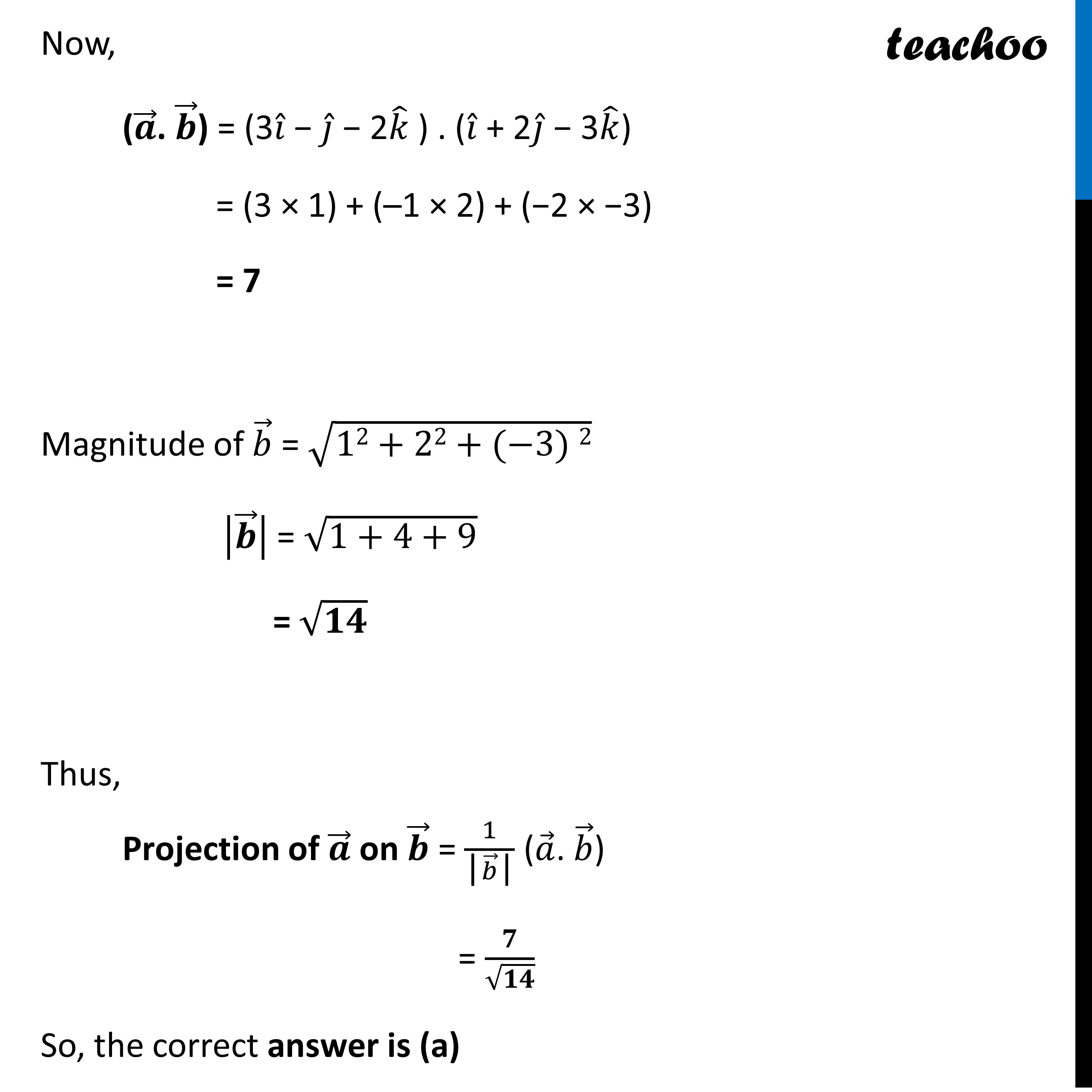CBSE Class 12 Sample Paper for 2023 Boards

Class 12
Solutions of Sample Papers and Past Year Papers - for Class 12 Boards

## (d) 7/2Learn in your speed, with individual attention - Teachoo Maths 1-on-1 Class

### Transcript

Question 8 The scalar projection of the vector 3𝑖 ̂−𝑗 ̂−2𝑘 ̂ 𝑜𝑛 𝑡ℎ𝑒 𝑣𝑒𝑐𝑡𝑜𝑟 𝑖 ̂+2𝑗 ̂−3𝑘 ̂ is (a) 7/√14 (b) 7/14 (c) 6/13 (d) 7/2 Let a = 3𝑖 ̂ − 𝑗 ̂ − 2𝑘 ̂ and b = 𝑖 ̂ + 2𝑗 ̂ − 3𝑘 ̂ We need to find Projection of 𝑎 ⃗ on 𝑏 ⃗ We know that Projection of 𝑎 ⃗ on 𝑏 ⃗ = 𝟏/("|" 𝒃 ⃗"|" ) (𝒂 ⃗. 𝒃 ⃗) Now, (𝒂 ⃗. 𝒃 ⃗) = (3𝑖 ̂ − 𝑗 ̂ − 2𝑘 ̂ ) . (𝑖 ̂ + 2𝑗 ̂ − 3𝑘 ̂) = (3 × 1) + (–1 × 2) + (−2 × −3) = 7 Magnitude of 𝑏 ⃗ = √(12+22+(−3) 2) |𝒃 ⃗ | = √(1+4+9) = √𝟏𝟒 Thus, Projection of 𝒂 ⃗ on 𝒃 ⃗ = 1/("|" 𝑏 ⃗"|" ) (𝑎 ⃗. 𝑏 ⃗) = 𝟕/√𝟏𝟒 So, the correct answer is (a)